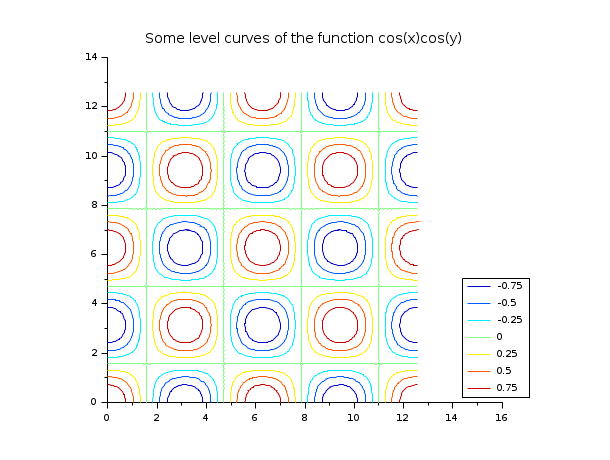Change language to:
English - 日本語 - Português - Русский
Aide de Scilab >> Graphiques > 2d_plot > contour2d

# contour2d

level curves of a surface on a 2D plot

### Syntax

```contour2d(x, y, z, nz, [style, strf, leg, rect, nax])
contour2d(x, y, z, nz, <opt_args>)```

### Arguments

x, y

two real row vectors of size `n1` and `n2`: the grid.

z

real matrix of size `(n1,n2)`, the values of the function on the grid or a Scilab function which defines the surface `z=f(x,y)`.

nz

the level values or the number of levels.

If `nz` is an integer

its value gives the number of level curves equally spaced from `zmin` to `zmax` as follows:

`z= zmin + (1:nz)*(zmax-zmin)/(nz+1)`Note that the `zmin` and `zmax` levels are not drawn (generically they are reduced to points) but they can be added with

```[im,jm] = find(z == zmin); // or zmax
plot2d(x(im)',y(jm)',-9,"000")```
If `nz` is a vector

`nz(i)` gives the value of the `i`-th level curve.

<opt_args>

This represents a sequence of statements `key1=value1, key2=value2`,... where `key1`, `key2`,... can be one of the following: `style`, `leg`, `rect`, `nax`, `strf` or `axesflag` and `frameflag` (see plot2d)

style, strf, leg, rect, nax

see plot2d. The argument `style` gives the dash styles or colors which are to be used for level curves. It must have the same size as the number of levels.

### Description

`contour2d` draws level curves of a surface `z=f(x,y)` on a 2D plot. The values of `f(x,y)` are given by the matrix `z` at the grid points defined by `x` and `y`.

You can change the format of the floating point number printed on the levels by using `xset("fpf",string)` where `string` gives the format in C format syntax (for example `string="%.3f"`). Use `string=""` to switch back to default format and use `string=" "` to suppress printing. This last feature is useful in conjunction with legends to display the level numbers in a legend and not directly onto the level curves as usual (see Examples).

The optional arguments `style`, `strf`, `leg`, `rect`, `nax` can be passed by a sequence of statements ```key1=value1, key2=value2``` ,... where keys may be `style`, `strf`, `leg`, `rect`, `nax`. In this case, the order has no special meaning.

Use `contour` to draw levels curves on a 3D surface.

### Examples

`contour2d(1:10,1:10,rand(10,10),5,rect=[0,0,11,11])````clf()
// changing the format of the printing of the levels
xset("fpf","%.2f")
contour2d(1:10,1:10,rand(10,10),5,rect=[0,0,11,11])``````// now an example with level numbers drawn in a legend
// Caution there are a number of tricks...
x = linspace(0,4*%pi,80);
z = cos(x')*cos(x);
clf(); f=gcf();
xset("fpf"," ")
// trick 1: this implies that the level numbers are not
//          drawn on the level curves
f.color_map=jetcolormap(7);
// trick 2: to be able to put the legend on the right without
//          interfering with the level curves use rect with a xmax
//          value large enough
contour2d(x,x,z,-0.75:0.25:0.75,frameflag=3,rect=[0,0,5*%pi,4*%pi])
// trick 3: use legends (note that the more practical legend function
//          will not work as soon as one of the level is formed by 2 curves)
legends(string(-0.75:0.25:0.75),1:7,"lr");
xtitle("Some level curves of the function cos(x)cos(y)")```